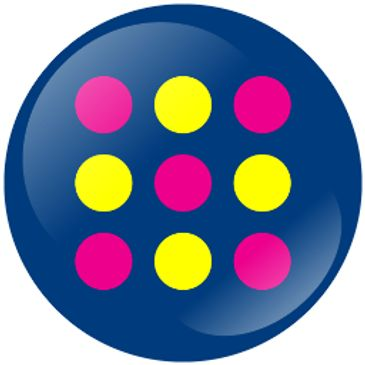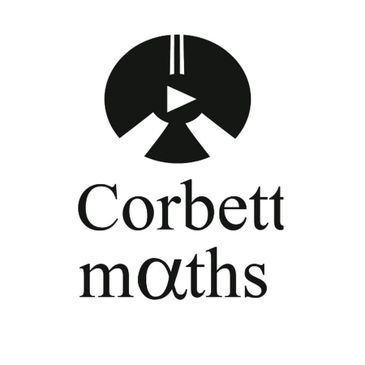#### Key Stage Three

At Key Stage 3, students follow a scheme of work based on the following mathematical processes and applications:

• Number.
• Algebra.
• Ratio.
• Proportion and rates of change.
• Geometry and measures.
• Probability.
• Statistics.

Students are taught in mixed ability classes for the first half term of Year 7 and then placed in ability groups which allows for the most able to work at a faster pace and be encouraged to think independently and the students who need more support can then work in smaller groups with help from Learning Support Assistants.

Students also have the opportunity to participate in the UKMT Mathematics Challenge at Junior Level (Years 7 and 8) and / or as part of the Team (Years 8 and 9).

#### Key Stage Four

At Key Stage 4, students study for the Edexcel GCSE.  The GCSE Mathematics examination is taken at Higher or Foundation Level.

In the Higher tier, grades are awarded from 4 to 9; in the Foundation tier, grades are awarded from 1 to 5.

As with Key Stage 3, the students follow a scheme of work based on the following mathematical processes and applications:

• Number.
• Algebra.
• Ratio.
• Proportion and rates of change.
• Geometry and measures.
• Probability.
• Statistics.

Students also have the opportunity to participate in the UKMT Mathematics Challenge at Intermediate Level (Years 9 to 11) and Mathematics Feast Days as part of a team (Year 10).

## Maths Revision Resources#### Edexcel Maths Specification#### Edexcel Maths Specification#### Corbett Maths#### Corbett Maths